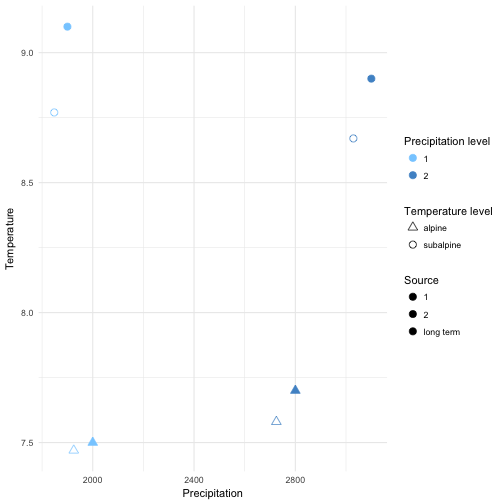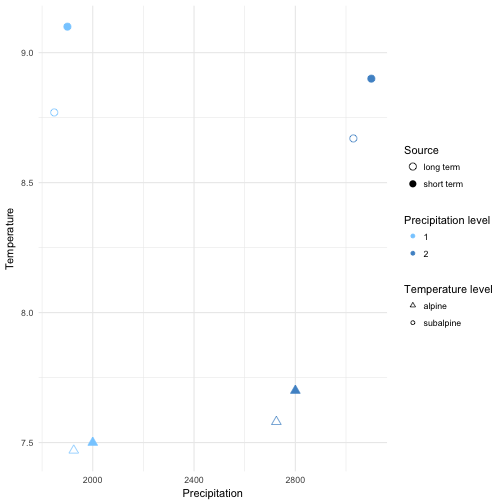# ggplot with colour, shape and colour depending fill

Everything is possible with ggplot in R. I realized that again today when plotting some climate data with different colour, shapes and fill. Color showed different precipitation levels, shape showed different temperature levels and I wanted filled symbols for the short term data and filled symbols for the long term data set. The complication was that the filled symbol depended as well on the precipitation level.

My solution was to manually fit different colours for fill, but this messed up the legend. So here comes trick number 2 to manually change the legend.

Let’s have a look at the plots and create a data set. There are 2 climate variables: temperature and precipitation. A factor for temperature and precipitaion with each 2 levels to define color and shape. And the source of the data (short or long term data).

``````# create a data set
Data <- data_frame(Temperature = c(8.77, 8.67, 7.47, 7.58, 9.1, 8.9, 7.5, 7.7),
Precipitation = c(1848, 3029, 1925, 2725, 1900, 3100,
2000, 2800),
Temperature_level = as.factor(c(rep("subalpine", 2),
rep("alpine", 2),
rep("subalpine", 2),
rep("alpine", 2))),
Precipitation_level = as.factor(c(rep(c(1,2),4))),
Source = c(rep("long term", 4), rep("short term", 4)))
``````

Let’s plot the data using ggplot. We want the filled symbol to be according to the precipitation level. So we use a ifelse statement for fill. If the source is the short term data, then use the precipitation colours, otherwise not. And manually we define the two blue colours and white for the symbols we do not want to have filled.

``````p <- ggplot(Data, aes(x = Precipitation, y = Temperature,
color = Precipitation_level,
shape = Temperature_level,
fill = factor(ifelse(Source == "short term",
Precipitation_level, Source)))) +
scale_color_manual(name = "Precipitation level",
values = c("skyblue1", "steelblue3")) +
scale_shape_manual(name = "Temperature level", values = c(24, 21)) +
# manually define the fill colours
scale_fill_manual(name = "Source",
values = c("skyblue1", "steelblue3", "white")) +
theme_minimal()
p + geom_point(size = 3)
``````The colours, shape and fill was plotted correctly, but this trick messed up the legend for the data source. The reason is that fill has 3 levels: 2 precipitation levels and one level for the long term data, which we coloured white.

We need another trick to fix this. We will use another factor with 2 levels and then replace the fill legend. First, we add different size for Source. It can be marginally different or have exacly the same value. This seems silly, but it’s useful to change the legend for fill. For changing the legend “guides” is a useful function. First we remove the fill legend. Then we use size which only has 2 levels and use override to draw different shapes for the two levels. And these shapes represent the filled and unfilled symbols.

``````p +